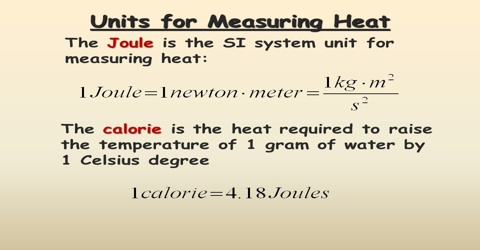# Heat in Thermodynamics

Heat is an important form of energy. It is difficult to define heat energy by a simple statement but the feeling of hotness or coldness is a common experience of all. Heat energy is capable of raising the temperature of a body. Temperature gives a feeling of how hot or how cold an object is. Temperature also determines the tendency of an object either to lose or gain heat. If an object A feels hotter than B. then object A has higher temperature than object B. If A and B are placed in contact with each other, heat will flow from object A to object B until the two objects are in thermal equilibrium and are at the same temperature. We say that heat can flow from a system at higher temperature to another system at lower temperature. From our experience we know that heat never flows from a body at a lower temperature to a body at a higher temperature. Heat energy can be partially converted into work. Other forms of energy can he completely converted into heat.

Unit of heat: The unit of heat is the caloric. A calorie is defined as the quantity of heat required to raise the temperature of 1.0 g of water through l.00 C. 1 calorie = 4.18 Joules. Sometimes heat is expressed in kilocalories (kcal), where 1 kcal = 1000 calories.

In the SI unit Joule is used as the unit of heat. 1000 Joules = 1 kilo Joule (KJ).

Heat energy can either be gained by the system or lost from it whenever there is a change in the state of the system. The amount of heat (q) gained or lost is directly proportional to the mass, m, of the system and the change in temperature.

q ∞ M (Tf – Ti)

The constant of proportionality is called the specific heat of the substance.

Here Ti and Tf are the initial and final temperatures respectively of the system. So,

∆T = (Tf – Ti)

Hence, q = (sp heat) m ∆T

If ∆T is positive, q is positive. This means heat energy has been gained by the system and the process is endothermic. If, however, ∆T is negative, q is also negative. In other words heat energy has been lost by the system. The process is exothermic.